••••# TI-Nspire CX CAS

Kč4,790.00
Tax included

One of the best graphics calculators to buy. CAS or Computer Algerbra System allows symbolic calculations, in other words, the result can be variable.

Quantity
IN STOCKStone shop is a guarantee of our e-shop.Overview of carriers and their price list.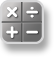Calculator
Perform computations and enter expressions, equations and formulas in proper math notation.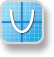Graphs
Plot and explore functions, equations and inequalities, animate points on objects and graphs, use sliders to explain their behavior and more.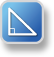Geometry
Construct and explore geometric figures and create animations.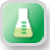Vernier DataQuest™ Application
Create a hypothesis graphically and replay data collection experiments all in a single application.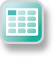Perform mathematical operations on data and visualize the connections between the data and their plots.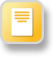Notes
Enter notes, steps, instructions and other comments on the screen alongside the math.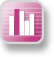Data & Statistics
Summarize and analyze data using different graphical methods such as histograms, box plots, bar and pie charts and more.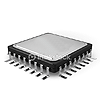#### Hardware

• Color, backlit display
• Screen size: 320 x 240 pixels (3.2" diagonal)
• Screen resolution: 125 DPI; 16-bit color
• USB port for computer connectivity, unit-to-unit communication with other TI-Nspire family handhelds.
• 100 MB storage memory / 64MB operating memory#### Built-in Functionality

• Computer Algebra System (CAS) capabilities
• Function, parametric, polar, sequence, and 3D graphing
• Multiple graphing functions can be defined, saved, graphed and analyzed at one time
• User-defined list names in Lists & Spreadsheet
• Eleven interactive zoom features
• Numeric evaluations in table format for all graphing modes
• Interactive analysis of function values, roots, maximums, minimums, integrals and derivatives
• Six different graph styles and 15 colors to select from for differentiating the look of each graph drawn
• Matrix operations: transpose and augment matrices; use elementary row operations; find inverse, determinant and reduced row echelon forms; convert matrices to lists and vice versa; calculate eigenvalues and eigenvectors
• List-based one- and two-variable statistical analysis, including logistic, sinusoidal, median-median, linear, logarithmic, exponential, power, quadratic polynomial, cubic polynomial, and quartic polynomial regression models
• Three statistical plot definitions for scatter plots, xy-line plots, histograms, regular and modified box-and-whisker plots, and normal probability plots
• Advanced statistics analysis, including 10 hypothesis testing functions, seven confidence interval functions and one-way analysis of variance
• Eighteen probability distributions functions, including the cumulative distribution function (CDF), probability density function (PDF) and inverse probability distribution function for normal, chi-squared, t-, and F- distributions; and the CDF and PDF for binomial, geometric and Poisson distributions
• Built-in interactive geometry capabilities
• Quick alpha keys
• Simple drop-down menus – emulate the intuitive operation of familiar computer features
• TI-Nspire™ Documents (.tns files) — compatible with TI-Nspire™ Software, TI-Nspire™ handhelds and TI-Nspire™ Apps for iPad® — can be created, edited, saved and reviewed
• Use images (.jpeg, .jpg, .bmp, .png formats) that can be overlaid with graphical elements
• Explore mathematical expressions in symbolic form, see patterns and understand the math behind the formulas
• Visualize of multiple representations of a single problem algebraic, graphical, geometric, numeric and written
• Chem Box feature allows easy input chemical formulas and equations
• Manipulate linked representations of properties to instantly update the others and show meaningful connections without switching screens (e.g.. grab a graphed function and move it to see the effect on corresponding equations and data lists)#### Programming

• Features a dedicated programming environment as well as programming libraries for global access to user-defined functions & programs#### Connectivity

• Electronically upgradeable graphing handheld allows you to have the most up-to-date functionality
• USB Cable included
• TI-ComputerLink Software allow information to be transferred to and from a computer#### Data Collection Support

• Compatible with TI-Nspire Lab Cradle, Vernier EasyLink® USB Sensor and Vernier EasyTemp® temperature sensor systems to allow collection and analysis of real-world data#### Accessories

• TI Rechargeable Battery
• Color slide cases available
• TI-Nspire™ CX Docking Station#### Support

• One-year limited warranty
• Toll-free help hotline: 1-800-TI-CARES
• Email: ti-cares@ti.com
• Classroom activities available
• Workshop Loan Program - you can borrow TI calculators for evaluation or workshops
• TI Technology Rewards program - accumulate points redeemable for additional TI products and services
• omplete guidebooks available
1181

Data sheet

Model of calculator
Graphic calculator
Number of functions
more than 1000
CAS (Computer Algebra System)
YES
Programmable
YES
Matrix
YES